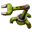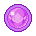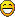## [0.17.48] [Modding] Construction bot animation: crash when using repeat_count

This subforum contains all the issues which we already resolved.
Smart InserterPosts: 2393
Joined: Fri Nov 06, 2015 7:41 pm

### [0.17.48] [Modding] Construction bot animation: crash when using repeat_count

Hi,

Construction bots have three sets of animations - idle, in_motion, working - and a complementary shadow version of each.

In vanilla, idle and in_motion are put together on the same sprite sheet, with the 16 different directions horizontally, and idle on in the first row and in_motion on the second row. working, however, has two frames per direction, so it is arranged in two vertical columns of directions instead. The shadows are on just one sheet but re-used for all three states - this means some finessing has to be done to make the arrangement compatible with the different layouts in the main sheets.

I've been trying to use a different arrangement which was supposed to be a lot simpler and consistent. I have two sheets, one for idle and in_motion (the bots are so slow they don't need to tilt in flight, but I may make a third sheet for in_motion later), and one for working. Both sheets have the direction as rows and animation frames as columns, like vanilla's working spritesheet does. The animation frames make it look like the bot's propeller is spinning. The advantage of doing this is that the sprite sheet layout is identical for all the bot's states and the Blender workflow is easier.

I ran into two problems, making two separate reports, this is the first:

I tried having just a single column for the shadows, setting frame_count to 1 and repeat_count to 2 (so that the number of frames matches between shadow and non-shadow - data validation won't let the game load otherwise). The game loads this definition just fine, but crashes hard when the time comes to render a shadow_working (log attached).

Prototype code that eventually results in this crash:

Code: Select all

``````{
cargo_centered = {
0,
0.2
},
collision_box = {
{
0,
0
},
{
0,
0
}
},
construction_vector = {
0.3,
0.22000000000000002
},
energy_per_move = "2.5kJ",
energy_per_tick = "0.025kJ",
flags = {
"placeable-player",
"player-creation",
"placeable-off-grid",
"not-on-map"
},
icon_size = 64,
idle = {
animation_speed = 1,
direction_count = 16,
frame_count = 2,
height = 80,
line_length = 2,
priority = "high",
scale = 0.5,
shift = {
0,
0
},
width = 80,
x = 0,
y = 0
},
in_motion = {
animation_speed = 1,
direction_count = 16,
frame_count = 2,
height = 80,
line_length = 2,
priority = "high",
scale = 0.5,
shift = {
0,
0
},
width = 80,
x = 0,
y = 0
},
max_energy = "0.75MJ",
max_health = 75,
max_to_charge = 0.95,
min_to_charge = 0.2,
minable = {
mining_time = 0.1,
result = "steambot"
},
name = "steambot",
resistances = {
{
percent = 85,
type = "fire"
}
},
selection_box = {
{
-0.5,
-0.5
},
{
0.5,
-0.5
}
},
animation_speed = 1,
direction_count = 16,
frame_count = 1,
height = 80,
line_length = 1,
priority = "high",
repeat_count = 2,
scale = 0.5,
shift = {
1,
0.5
},
width = 80,
x = 0,
y = 0
},
animation_speed = 1,
direction_count = 16,
frame_count = 1,
height = 80,
line_length = 1,
priority = "high",
repeat_count = 2,
scale = 0.5,
shift = {
1,
0.5
},
width = 80,
x = 0,
y = 0
},
animation_speed = 1,
direction_count = 16,
frame_count = 1,
height = 80,
line_length = 1,
priority = "high",
repeat_count = 2,
scale = 0.5,
shift = {
1,
0.5
},
width = 80,
x = 0,
y = 0
},
smoke = {
animation_speed = 0.3,
filename = "__base__/graphics/entity/smoke-construction/smoke-01.png",
frame_count = 19,
height = 32,
line_length = 19,
shift = {
0.078125,
-0.15625
},
width = 39
},
sparks = {
{
animation_speed = 0.3,
filename = "__base__/graphics/entity/sparks/sparks-01.png",
frame_count = 19,
height = 34,
line_length = 19,
shift = {
-0.109375,
0.3125
},
tint = {
a = 1,
b = 0,
g = 0.9,
r = 1
},
width = 39
},
{
animation_speed = 0.3,
filename = "__base__/graphics/entity/sparks/sparks-02.png",
frame_count = 19,
height = 32,
line_length = 19,
shift = {
0.03125,
0.125
},
tint = {
a = 1,
b = 0,
g = 0.9,
r = 1
},
width = 36
},
{
animation_speed = 0.3,
filename = "__base__/graphics/entity/sparks/sparks-03.png",
frame_count = 19,
height = 29,
line_length = 19,
shift = {
-0.0625,
0.203125
},
tint = {
a = 1,
b = 0,
g = 0.9,
r = 1
},
width = 42
},
{
animation_speed = 0.3,
filename = "__base__/graphics/entity/sparks/sparks-04.png",
frame_count = 19,
height = 35,
line_length = 19,
shift = {
-0.0625,
0.234375
},
tint = {
a = 1,
b = 0,
g = 0.9,
r = 1
},
width = 40
},
{
animation_speed = 0.3,
filename = "__base__/graphics/entity/sparks/sparks-05.png",
frame_count = 19,
height = 29,
line_length = 19,
shift = {
-0.109375,
0.171875
},
tint = {
a = 1,
b = 0,
g = 0.9,
r = 1
},
width = 39
},
{
animation_speed = 0.3,
filename = "__base__/graphics/entity/sparks/sparks-06.png",
frame_count = 19,
height = 36,
line_length = 19,
shift = {
0.03125,
0.3125
},
tint = {
a = 1,
b = 0,
g = 0.9,
r = 1
},
width = 44
}
},
speed = 0.03,
speed_multiplier_when_out_of_energy = 0.2,
transfer_distance = 0.5,
type = "construction-robot",
working = {
animation_speed = 1,
direction_count = 16,
frame_count = 2,
height = 80,
line_length = 2,
priority = "high",
scale = 0.5,
shift = {
0,
0
},
width = 80,
x = 0,
y = 0
},
working_light = {
color = {
b = 0.4,
g = 0.7,
r = 0.8
},
intensity = 0.8,
size = 3
},
working_sound = {
audible_distance_modifier = 0.5,
max_sounds_per_type = 3,
probability = 0.0055555555555555554,
sound = {
{
filename = "__base__/sound/flying-robot-1.ogg",
volume = 0.6
},
{
filename = "__base__/sound/flying-robot-2.ogg",
volume = 0.6
},
{
filename = "__base__/sound/flying-robot-3.ogg",
volume = 0.6
},
{
filename = "__base__/sound/flying-robot-4.ogg",
volume = 0.6
},
{
filename = "__base__/sound/flying-robot-5.ogg",
volume = 0.6
}
}
}
}
``````
Sorry but I can't post the mod that produces this - I can provide it to a dev in private if they need it.
Attachments
factorio-current.log
"If Stalin had a good writeup on programming, would linking that be dangerous?"

posila
Factorio StaffPosts: 5147
Joined: Thu Jun 11, 2015 1:35 pm
Contact:

### Re: [0.17.48] [Modding] Construction bot animation: crash when using repeat_count

Thanks for the reportMy fix for 71909 was to use repeat_count, which crashed for me, so I had to fixed this crash to be able to fix 71909Since you made this detailed bug report, I'll put it to the changelog too, thanks.

Fixed for 0.17.49

Smart InserterPosts: 2393
Joined: Fri Nov 06, 2015 7:41 pm

### Re: [0.17.48] [Modding] Construction bot animation: crash when using repeat_count

posila wrote:
Wed Jun 12, 2019 9:49 am
Thanks for the reportMy fix for 71909 was to use repeat_count, which crashed for me, so I had to fixed this crash to be able to fix 71909Since you made this detailed bug report, I'll put it to the changelog too, thanks.

Fixed for 0.17.49
Zwei dumme, ein gedanke ...

Thanks for this, really appreciated.
"If Stalin had a good writeup on programming, would linking that be dangerous?"